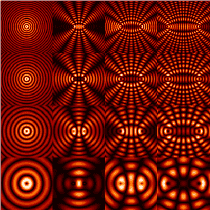# How does a standing wave form?

Summary:
A short explanation on how standing waves form would be appreciated
I understand how waves undergo superposition. However, for a standing wave, the reflected wave is a mirror opposite of the incoming wave. By the superposition principle, won’t the 2 waves add up to 0, at all points?

•Delta2

etotheipi
Take, for instance, ##f(x,t) = \sin{(kx - \omega t)}## and ##g(x,t) = \sin{(kx + \omega t)}##; by a trig identity then$$h(x,t) := f(x,t) + g(x,t) = 2 \sin{(kx)} \cos{(\omega t)}$$which is a standing wave, and definitely not everywhere zero! It's because the incident and reflected waves generally don't meet in anti-phase, apart from the times when the wave-crests meet the boundary [for the above example you can take the boundary, or mirror, to be ##x=0##].

Try plotting some oppositely travelling waves on https://www.desmos.com/calculator to build intuitionLast edited by a moderator:
•hutchphd, vanhees71, Delta2 and 2 others
kuruman
Homework Helper
Gold Member
When two waves of the same frequency and wavelength travel in opposite directions, something cancels. It’s not the waves themselves (that’s destructive interference) it’s the direction of travel hence the name “standing wave” as opposed to “traveling wave”.

•phantomvommand, etotheipi and Delta2
robphy
Homework Helper
Gold Member
There are instants when the superpositioned waves are zero (namely when $t$ is such that @etotheipi 's $\cos(\omega t)=0$), but apart from those instants, only the "nodes" (the $x$'s where $\sin(kx)=0$) of the standing wave are forever zero--the superpositioned waves for other locations have a nonzero displacement.

•phantomvommand and etotheipi
sophiecentaur
Gold Member
2020 Award
It’s not the waves themselves (that’s destructive interference)
Why distinguish between the situations. If the resultant amplitude is less than the mean of one wave then you have destructive interference. The standing wave pattern is stationary in just the same way that a (stationary / standing) interference pattern forms on a screen. Precisely the same phenomenon.
Many standing wave patterns do not have perfect zeros, of course.

•phantomvommand
Mister T
Gold Member
However, for a standing wave, the reflected wave is a mirror opposite of the incoming wave.
I don't know what you mean by a mirror opposite. There are instants when the reflected wave is identical in shape to the incoming wave. In those cases you get constructive interference. There are other instants where they are out of phase and you get destructive interference.

•phantomvommand
Summary:: A short explanation on how standing waves form would be appreciated

I understand how waves undergo superposition. However, for a standing wave, the reflected wave is a mirror opposite of the incoming wave. By the superposition principle, won’t the 2 waves add up to 0, at all points?
That rather depends on the mirror!

In some cases, yes this is precisely what happens and it is called a 'perfectly matched load'.

In others, there is a mismatch between the incoming wave and the 'load'.

That's why in RF engineering, for example, if one wants to be rid of standing waves (which one very much usually does want) then the transmission line and the load it goes into have to be configured in such a way that their impedances are conjugate.

In mechanical examples, such as a plucked string on a guitar, the mechanical wave of the string has a low 'mechanical' impedance and the bridge it is attached to is very high, hence the reflected wave. (If the bridge was made of a sponge then it'd be more a matched load and the energy absorbed.) This impedance mismatch creates the reflection but it is imperfect and some small component of the energy in that wave is dissipated into the bridge, the remainder goes back into the string. That wave resonates on the string, and the small component of the energy that goes into the bridge (defined by the 'Q' of the system) then stimulates modes in the guitar body which radiates the noise.

•Delta2, sophiecentaur and phantomvommand
sophiecentaur
Gold Member
2020 Award
Summary:: A short explanation on how standing waves form would be appreciated

By the superposition principle, won’t the 2 waves add up to 0, at all points?
To get cancellation, the waves have to be of the same amplitude and exactly in anti phase. The phase of the forward wave, viewed at one point, will constantly be progressing. The phase of the reflected wave will also be constantly progressing. It's only if the path from the wave, via the mirror and back, is exactly a whole number of wavelengths that the phases of the two waves are in anti phase to give a node. (Both in phase for an antinode) at nowhere else will this happen and you will get a non-zero resultant of the complex addition of the two waves. If you assume a rigid fixing at the 'mirror' or a short circuit if it's a electrical wave then the reflected signal will be in anti phase at the point of reflection and if you have a free end or an open circuit at the reflection point, the reflected wave will be in phase and the positions of nodes and antinodes in the standing wave will swap.

•Delta2 and phantomvommand
sophiecentaur